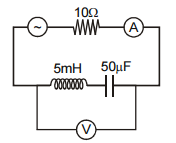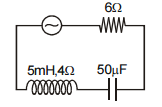Select Chapter Topics:# An AC ammeter is used to measure the current in a circuit. When a given direct current passes through the circuit, the ac ammeter reads 6 A. When another alternating current passes through the circuit, the AC ammeter reads 8 A. Then the reading of this ammeter if DC and AC flow through the circuit simultaneously is: 1. $10\sqrt{2}$ A 2. 14 A 3. 10 A 4. 15 ASubtopic:  AC vs DC |
65%
From NCERT
To view explanation, please take trial in the course.
NEET 2023 - Target Batch - Aryan Raj Singh
Hints
To view explanation, please take trial in the course.
NEET 2023 - Target Batch - Aryan Raj Singh

If q is the capacitor's charge and i is the current at time t, the voltage V will be:1 $$\mathrm{L} \frac{\mathrm{di}}{\mathrm{dt}}+\mathrm{iR}-\frac{\mathrm{q}}{\mathrm{C}}=\mathrm{V}$$ 2 $$\mathrm{L} \frac{\mathrm{di}}{\mathrm{dt}}-\mathrm{iR}+\frac{\mathrm{q}}{\mathrm{C}}=\mathrm{V}$$ 3 $$\mathrm{L} \frac{\mathrm{di}}{\mathrm{dt}}+\mathrm{iR}+\frac{\mathrm{q}}{\mathrm{C}}=\mathrm{V}$$ 4 $$\mathrm{L} \frac{\mathrm{di}}{\mathrm{dt}}-\mathrm{iR}-\frac{\mathrm{q}}{\mathrm{C}}=\mathrm{V}$$Subtopic:  Different Types of AC Circuits |
53%
From NCERT
Hints

A direct current of 5 A is superimposed on an alternating current I = 10$\mathrm{sin}\omega t$ flowing through a wire. The effective value of the resulting current will be:

1. $$15/2~A$$

2.

3.

4. 15 ASubtopic:  AC vs DC |
60%
From NCERT
Hints

An L-C-R series circuit with 100 Ω resistance is connected to an AC source of 200 V and an angular frequency of 300 rad/s. When only the capacitance is removed, the current lags behind the voltage by $60°$. When only the inductance is removed, the current leads the voltage by $60°$. Calculate the power dissipated in the L-C-R circuit.

1. 200 W

2. 400 W

3. 300 W

4. ZeroSubtopic:  Power factor |
64%
From NCERT
To view explanation, please take trial in the course.
NEET 2023 - Target Batch - Aryan Raj Singh
Hints
To view explanation, please take trial in the course.
NEET 2023 - Target Batch - Aryan Raj Singh

An ideal resistance R, ideal inductance L, ideal capacitance C, and AC voltmeters ${\mathrm{V}}_{1},$ ${\mathrm{V}}_{2},$ ${\mathrm{V}}_{3}$ $\mathrm{and}$ ${\mathrm{V}}_{4}$ are connected to an AC source as shown. At resonance:1 Reading in $$V_3$$ = Reading in $$V_1$$ 2 Reading in $$V_1$$ = Reading in $$V_2$$ 3 Reading in $$V_2$$ = Reading in $$V_4$$ 4 Reading in $$V_2$$ = Reading in $$V_3$$Subtopic:  Different Types of AC Circuits |
84%
From NCERT
To view explanation, please take trial in the course.
NEET 2023 - Target Batch - Aryan Raj Singh
Hints
To view explanation, please take trial in the course.
NEET 2023 - Target Batch - Aryan Raj Singh

A transistor-oscillator using a resonant circuit with an inductance L (of negligible resistance) and a capacitance C has a frequency f. If L is doubled and C is changed to 4C, the frequency will be:

1. f/4

2. 8f

3. f/(2√2)

4. f/2Subtopic:  Different Types of AC Circuits |
86%
From NCERT
AIPMT - 2006
To view explanation, please take trial in the course.
NEET 2023 - Target Batch - Aryan Raj Singh
Hints
To view explanation, please take trial in the course.
NEET 2023 - Target Batch - Aryan Raj Singh

In an L-C-R series AC circuit, the voltage across each of the components - L, C and R is 50 V. The voltage across the L-R combination will be:
1. 50 V
2. $$50 \sqrt{2} ~V$$
3. 100 V
4. 0 VSubtopic:  Different Types of AC Circuits |
69%
From NCERT
To view explanation, please take trial in the course.
NEET 2023 - Target Batch - Aryan Raj Singh
Hints
To view explanation, please take trial in the course.
NEET 2023 - Target Batch - Aryan Raj Singh

The AC source in the circuit shown in the figure produces a voltage V = 20cos(2000t) volts. Neglecting source resistance, the voltmeter and ammeter readings will be (approximately):1. 4 V, 2.0 A

2. 0 V, 2 A

3. 0 V, 1.4 A

4. 8 V, 2.0 ASubtopic:  Different Types of AC Circuits |
60%
From NCERT
To view explanation, please take trial in the course.
NEET 2023 - Target Batch - Aryan Raj Singh
Hints
To view explanation, please take trial in the course.
NEET 2023 - Target Batch - Aryan Raj Singh

In the circuit shown, the AC source has a voltage
V = 20 cos(ωt) volts with ω= 2000 rad/s. The amplitude of the current will be nearest to:1. 2 A

2. 3.3 A

3. 2/$\sqrt{5}$ A

4. $\sqrt{5}$ ASubtopic:  Different Types of AC Circuits |
56%
From NCERT
To view explanation, please take trial in the course.
NEET 2023 - Target Batch - Aryan Raj Singh
Hints
To view explanation, please take trial in the course.
NEET 2023 - Target Batch - Aryan Raj Singh

In a heating arrangement, an alternating current having a peak value of $$28~\mathrm{A}$$ is used. To produce the same heat energy, if direct current is used to produce the same amount of heat, then its magnitude will be:
1. about $$14~\mathrm{A}$$
2. about $$28~\mathrm{A}$$
3. about $$20~\mathrm{A}$$
4. cannot saySubtopic:  AC vs DC |
57%
From NCERT
To view explanation, please take trial in the course.
NEET 2023 - Target Batch - Aryan Raj Singh
Hints